# Addendum to Total Growth of Open Source Paper

Based on multiple requests, for the Total Growth of Open Source paper, we are providing a table of doubling times for the exponential models as well as semi-log scale graphs of the growth curves.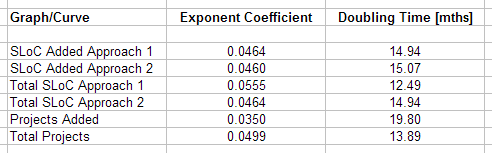Table 1: Doubling times for the growth curves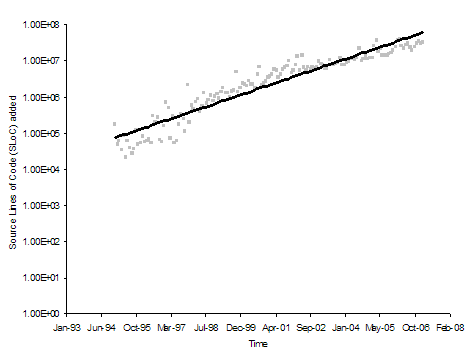Figure 1: Semi-log scale graph of source lines of code added (Approach 1)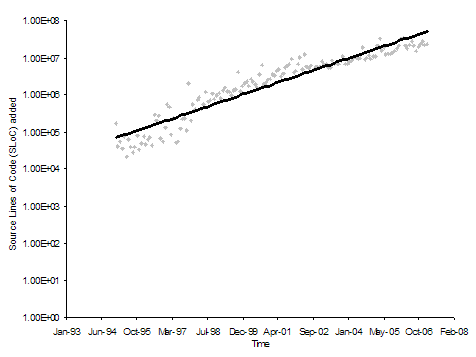Figure 2: Semi-log scale graph of source lines of code added (Approach 2)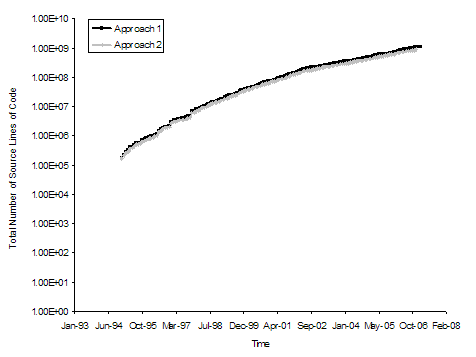Figure 3: Semi-log scale graph of total source lines of code (both approaches)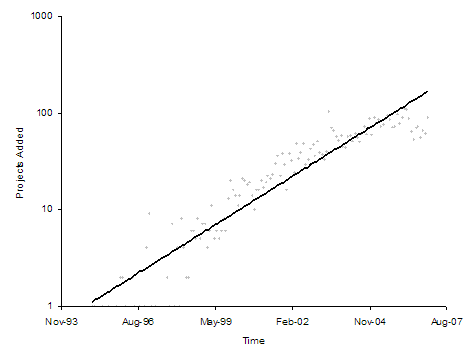Figure 4: Semi-log scale graph of number of open source projects added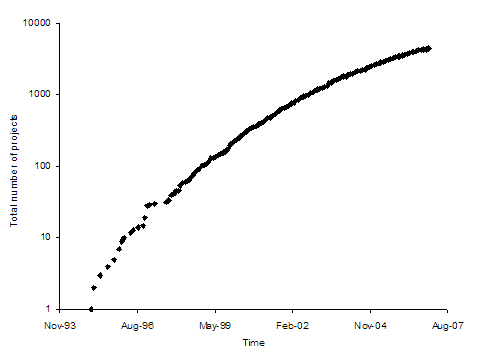Figure 5: Semi-log scale graph of total number of open source projects